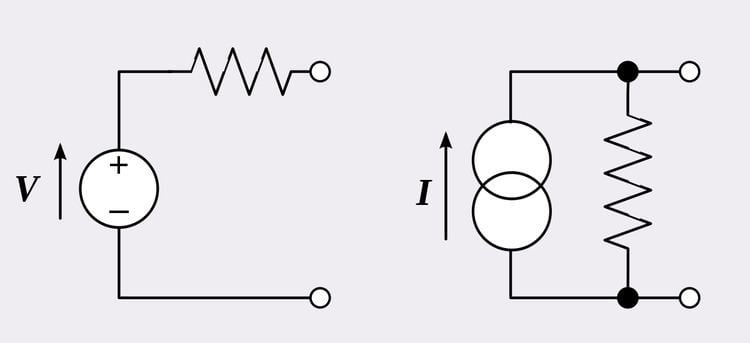# Generator (circuit theory)

Updated on
Covid-19A generator in electrical circuit theory is one of two ideal elements: an ideal voltage source, or an ideal current source. These are two of the fundamental elements in circuit theory. Real electrical generators are most commonly modelled as a non-ideal source consisting of a combination of an ideal source and a resistor. Voltage generators are modelled as an ideal voltage source in series with a resistor. Current generators are modelled as an ideal current source in parallel with a resistor. The resistor is referred to as the internal resistance of the source. Real world equipment may not perfectly follow these models, especially at extremes of loading (both high and low) but for most purposes they suffice.

The two models of non-ideal generators are interchangeable, either can be used for any given generator. Thévenin's theorem allows a non-ideal current source model to be converted to a non-ideal voltage source model and Norton's theorem allows a non-ideal voltage source model to be converted to a non-ideal current source model. Both models are equally valid, but the voltage source model is more applicable to when the internal resistance is low (that is, much lower than the load impedance) and the current source model is more applicable when the internal resistance is high (compared to the load).

## Symbols

Symbols commonly used for ideal sources are shown in the figure. Symbols do vary from region to region and time period to time period. Another common symbol for a current source is two interlocking circles.

## References

Similar Topics
Were in the Money (film)
Wayne Carleton
Mensa Otabil
Topics

 B i Link H2
 L